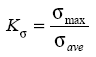Related Resources: material science

Stress Concentration Fundamentals

Stress concentration is defined as - Localized stress considerably higher than average (even in uniformly loaded cross sections of uniform thickness) due to abrupt changes in geometry or localized loading.

Stress concentration is often called "stress raisers" or "stress risers"

Geometric discontinuities cause localized stress increases above the average or far-field stress. A stress raiser's effect can be determined quantitatively in several ways, but not always readily. The simplest method, if applicable, is to use a known theoretical stress concentration factor, Kt, to calculate the peak stress from the nominal, or average, value;Equation 1

This is illustrated in Figure 1. The area under the true stress distribution always equals the area under the nominal stress level,Figure 1Stress distribution (simplistic) in a notched member under uniaxial load.

The factor Kt depends mainly on the geometry of the notch, not on the material, except when the material deforms severely under load. Kt values are normally obtained from plots such as in Figure 2 and arestrictly valid only for ideally elastic, stiff members. Kt values can also be determined by FEA or by several experimental techniques. There are no Kt values readily available for sharp notches and cracks, but one can always assume that such discontinuities produce the highest stress concentrations, sometimes factors of tens. This is the reason for brittle, high-strength materials being extremely sensitive even to minor scratches. In fatigue, for example, invisible toolmarks may lead to premature, unexpected failures in strong steels.Figure 2Figure 3 Samples of elastic stress concentration factors., Dowling, N. E. 1993. Mechanical Behavior of Materials. Prentice-Hall, Englewood Cliffs, NJ. With permission.)

There are many other factors that may seem similar to Kt, but they should be carefully distinguished. The first is the true stress concentration factor Ks, defined as:which means that Ks = Kt (by Equation 1) for ideally elastic materials. Ks is most useful in the case of ductile materials that yield at the notch tip and lower the stress level from that indicated by Kt. Similarly, a true strain concentration factor, Ke, is defined aswhere eave = save/E.

Furthermore, a large number of stress intensity factors are used in fracture mechanics, and these (such as K, Kc, KI, etc.) are easily confused with Kt and Ks, but their definitions and uses are different.

Concentration Factor for Cracks:

The maximum stress felt near a crack occurs in the area of lowest radius of curvature. In an elliptical crack of length 2a and width 2b, under an applied external stress s, the stress at the ends of the major axes is given by:where p is the radius of curvature of the crack tip. A stress concentration factor is the ratio of the highest stress (smax)) to a reference stress (s) of the gross cross-section. As the radius of curvature approaches zero, the maximum stress approaches infinity. Note that the stress concentration factor is a function of the geometry of a crack, and not of its size. These factors can be found in typical engineering reference materials to predict the stresses that could otherwise not be analyzed using strength of materials approaches. This is not to be confused with 'Stress Intensity Factor'.

Concentration factor calculation:

There are experimental methods for measuring stress concentration factor including photoelastic stress analysis, brittle coatings or strain gauges. While all these approaches have been successful, all also have experimental, environmental, accuracy and/or measurement disadvantages.

During the design phase, there are multiple approaches to estimating stress concentration factors. Several catalogs of stress concentration factors have been published. Theoretical approaches, using elasticity or strength of material considerations, can lead to equations similar to the one shown above.

There may be small differences between the catalog, FEM and theoretical values calculated. Each method has advantages and disadvantages. Many catalog curves were derived from experimental data. FEM calculates the peak stresses directly and nominal stresses may be easily found by integrating stresses in the surrounding material. The result is that engineering judgment may have to be used when selecting which data applies to making a design decision. Many theoretical stress concentration factors have been derived for infinite or semi-infinite geometries which may not be analyzable and are not testable in a stress lab, but tackling a problem using two or more of these approaches will allow an engineer to achieve an accurate conclusion.
• ESDU64001: Guide to stress concentration data (ISBN 1-86246-279-8)
• Pilkey, Walter D, Peterson's Stress Concentraton Factors, Wiley, 2nd Ed (1999). ISBN 0-471-53849-3
• American Institute of Steel Construction
• Mechanical Engineering Handbook, - Frank Kreith 1999